Olympiad Test Level 2: Logical Reasoning

# Olympiad Test Level 2: Logical Reasoning

Test Description

## 15 Questions MCQ Test GK Olympiad for Class 6 | Olympiad Test Level 2: Logical Reasoning

Olympiad Test Level 2: Logical Reasoning for Class 6 2022 is part of GK Olympiad for Class 6 preparation. The Olympiad Test Level 2: Logical Reasoning questions and answers have been prepared according to the Class 6 exam syllabus.The Olympiad Test Level 2: Logical Reasoning MCQs are made for Class 6 2022 Exam. Find important definitions, questions, notes, meanings, examples, exercises, MCQs and online tests for Olympiad Test Level 2: Logical Reasoning below.
Solutions of Olympiad Test Level 2: Logical Reasoning questions in English are available as part of our GK Olympiad for Class 6 for Class 6 & Olympiad Test Level 2: Logical Reasoning solutions in Hindi for GK Olympiad for Class 6 course. Download more important topics, notes, lectures and mock test series for Class 6 Exam by signing up for free. Attempt Olympiad Test Level 2: Logical Reasoning | 15 questions in 30 minutes | Mock test for Class 6 preparation | Free important questions MCQ to study GK Olympiad for Class 6 for Class 6 Exam | Download free PDF with solutions
 1 Crore+ students have signed up on EduRev. Have you?
Olympiad Test Level 2: Logical Reasoning - Question 1

### The difference of two numbers is 1365. On dividing the larger number by the smaller, we get 6 as quotient and the 15 as remainder. What is the smaller number?

Detailed Solution for Olympiad Test Level 2: Logical Reasoning - Question 1

Let the smaller number be x.
Then larger number = (x + 1365).
∴ x + 1365 = 6x + 15
⇒ 5x = 1350
⇒ x = 270
Smaller number = 270.

Olympiad Test Level 2: Logical Reasoning - Question 2

### On dividing a number by 5, we get 3 as the remainder. What will the remainder be when the square of this number is divided by 5?

Detailed Solution for Olympiad Test Level 2: Logical Reasoning - Question 2

Let the number be x and on dividing x by 5, we get k as quotient and 3 as remainder.
∴ x = 5k + 3
∴ x2 = (5k +3)2
= (25k2 + 30k + 9)
= 5(5k2 + 6k+1) + 4
∴ on dividing x2 by 5, we get 4 as remainder.

Olympiad Test Level 2: Logical Reasoning - Question 3

### From his house, Lokesh went 15 kms to the North. Then he turned West and covered 10 kms. Then he turned South and covered 5 kms. Finally, turning to East, he covered 10 kms. In which direction is he from his house?

Detailed Solution for Olympiad Test Level 2: Logical Reasoning - Question 3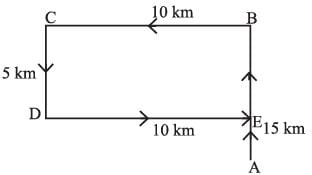Final position of Lokesh is at point ‘E’ which is north from his house.

Olympiad Test Level 2: Logical Reasoning - Question 4

Directions: Given here is a multiple bar diagram of the scores of four players in two innings. Study the diagram and answer the questions.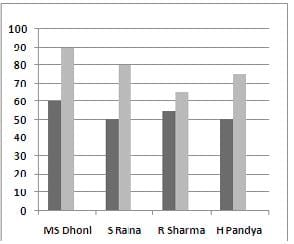The who scored highest average runs of two innings is:

Detailed Solution for Olympiad Test Level 2: Logical Reasoning - Question 4

Required average runs scored by MS Dhoni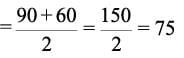Required average runs scored by S Raina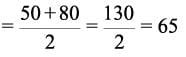Required average runs scored by R Sharma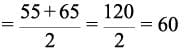Required average runs scored by H Pandya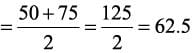The required cricketer is M.S. Dhoni.

Olympiad Test Level 2: Logical Reasoning - Question 5

Directions: Given here is a multiple bar diagram of the scores of four players in two innings. Study the diagram and answer the questions.The total scores in the first innings contributed by the four players is

Detailed Solution for Olympiad Test Level 2: Logical Reasoning - Question 5

Required total score = 60 + 50 + 55 + 50 = 215

Olympiad Test Level 2: Logical Reasoning - Question 6

Group the following figures into three classes using each figure only once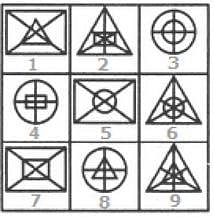Detailed Solution for Olympiad Test Level 2: Logical Reasoning - Question 6

1, 5, 7 contain a rectangle with its two diagonals as the outer element and another element (similar or different) placed inside it.
2, 6, 9 contain a triangle with its three medians as the outer element and another element (similar or different) placed inside it.
3, 4, 8 contain a circle with its two mutually perpendicular diameters as the outer element and another element (similar or different) placed inside it.

Olympiad Test Level 2: Logical Reasoning - Question 7

The least number which when divided by 5, 6, 7 and 8 leaves a remainder 3, but when divided by 9 leaves no remainder, is

Detailed Solution for Olympiad Test Level 2: Logical Reasoning - Question 7

L.C.M. of 5, 6, 7, 8 = 840.
Required number is of the form 840k + 3
Least value of k for which (840k + 3 ) is divisible by 9 is k = 2.
Required number = (840 x 2 + 3) = 1683.

Olympiad Test Level 2: Logical Reasoning - Question 8

In a mixture 60 litres, the ratio of milk and water 2 : 1. If the this ratio is to be 1 : 2, then the quantity of water to be further added is

Detailed Solution for Olympiad Test Level 2: Logical Reasoning - Question 8

Quantity of M ilk = 60 x (2/3) = 40 liters Quantity of water = 60 - 40 = 20 liters
As per question we need to add water to get quantity 1:2
⇒ 40/(20 + x) = 1/2
⇒ 20 + x = 80
⇒ x = 60 liters

Olympiad Test Level 2: Logical Reasoning - Question 9

A rectangular lawn is 120 m by 90 m and has two roads each 15m wide running in the middle of it. One parallel to its length and other parallel to the breadth. Find the cost of gravelling the road at ₹ 20 per sq meter.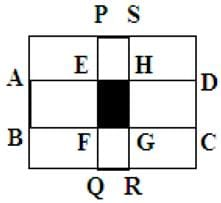Detailed Solution for Olympiad Test Level 2: Logical Reasoning - Question 9

According to question,
Area of road that is parallel to length
= 120 x 15 = 1800 sq m
Area of road that is parallel to breadth
= 90 x 15 = 1350 sqm
Area of intersection of two roads
= 15 x 15 = 22m2
∴ Area of two road = (1800+ 1350-225)
= 2925 sq m
Cost at 1 sq m = 20
So, Cost at 2925 sq m = 20 x 2925
= ₹ 58500

Olympiad Test Level 2: Logical Reasoning - Question 10

There are four prime numbers written in ascending order. The product of first three is 385 and that of last three is 1001. The last number is

Detailed Solution for Olympiad Test Level 2: Logical Reasoning - Question 10

According to question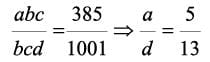so d = 13

Olympiad Test Level 2: Logical Reasoning - Question 11

A courtyard is 25 meters long and 16 meters board is to be paved with bricks of dimensions 20 cm by 10 cm. The total number of bricks required is :

Detailed Solution for Olympiad Test Level 2: Logical Reasoning - Question 11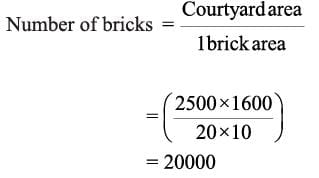Olympiad Test Level 2: Logical Reasoning - Question 12

A rectangular grassy plot is 112 m by 78 m. It has a gravel path 2.5 m wide all round it on the inside. Find the cost of constructing it at ₹ 2 per square meter?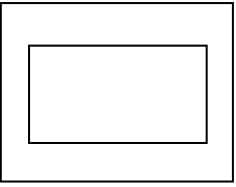Detailed Solution for Olympiad Test Level 2: Logical Reasoning - Question 12

Are of  path = 2 x (1 + b - 2x)
= 5 (112 + 78 - 5)
= 5 * 185 = 925 sq m
Therefore, Required cost = (925x2)
= ₹ 1850

Olympiad Test Level 2: Logical Reasoning - Question 13

Find the area of the shaded region in the figure: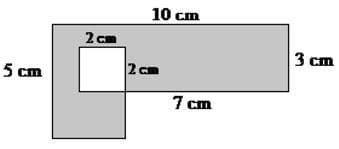Detailed Solution for Olympiad Test Level 2: Logical Reasoning - Question 13

Step 1: Findarea of inner square
= 2 cm x 2 cm = 4 cm2
Step 2 : Find area of outer shape
= (2 cm x 3 cm) + (10 cm x 3 cm)
= 6 cm2 + 30 cm2 = 36 cm2
Step 3 : Area of shaded region
= area of outer shape - area of inner square
= 36 cm2 - 4 cm2 = 32 cm2

Olympiad Test Level 2: Logical Reasoning - Question 14

A man buys an item at ₹ 1200 and sells it at the loss of 20 percent. Then what is the selling price of that item?

Detailed Solution for Olympiad Test Level 2: Logical Reasoning - Question 14

Here always remember, when ever, x% loss,
it means S.P. = (100 - x) % of C.P
When ever, x% profit, it means S.P. = (100 + x) % of C.P
So here will be (100 - x) % of C.P.
= 80 % of 1200
= 80/100 x 1200
= 960

Olympiad Test Level 2: Logical Reasoning - Question 15

Ram walks 2 km to the East, and then he turns to South and walks 6 km. He again turns to East and walks 2 km. Then he turns to North and walks 12 km. How far is he from the starting point?

Detailed Solution for Olympiad Test Level 2: Logical Reasoning - Question 15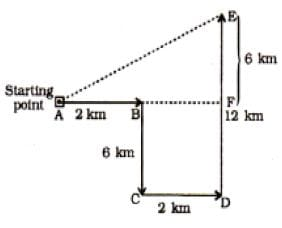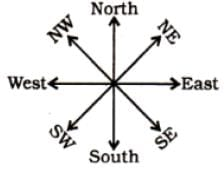Required distance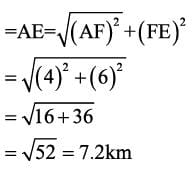## GK Olympiad for Class 6

22 docs|58 tests
 Use Code STAYHOME200 and get INR 200 additional OFF Use Coupon Code
Information about Olympiad Test Level 2: Logical Reasoning Page
In this test you can find the Exam questions for Olympiad Test Level 2: Logical Reasoning solved & explained in the simplest way possible. Besides giving Questions and answers for Olympiad Test Level 2: Logical Reasoning, EduRev gives you an ample number of Online tests for practice

22 docs|58 tests